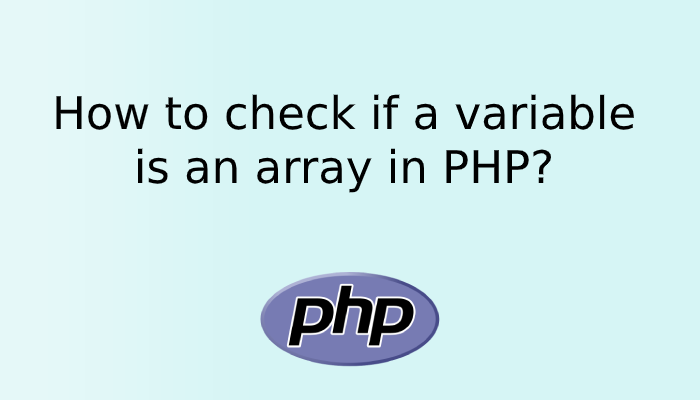# How to check if a variable is an array in PHP?Sometimes we need to check if the variable is an array or not. For example, we get value from the database or from anywhere in the application, before processing that value we need to do some checks like if the variable is an array then do something otherwise perform some other action. Basically it is to check if the input we are receiving is an array or not, so can use it as a conditional operator and make decisions as per requirement in the project.

In this post we will let you know, how you can check the variable is an array or not with the help of PHP inbuilt function.

PHP provides is_array() function which checks whether a variable is an array or not. This function returns true 1 if the variable is an array, otherwise, it returns false on nothing. Let’s understand the same as the below example.

## Syntax

``is_array(variable);``
```<?php
\$x = "Hello";
echo "x is " . is_array(\$x) . "<br>";

\$y = array("red", "green", "blue");
echo "y is " . is_array(\$y) . "<br>";

\$z = array("Peter"=>"35", "Ben"=>"37", "Joe"=>"43");
echo "z is " . is_array(\$z) . "<br>";

\$v = "red, green, blue";
echo "v is " . is_array(\$v) . "<br>";
?>```

Output :-

``````x is
y is 1
z is 1
v is``````

In the first, we have \$x with a string value. Now when we put this value in is_array() function is returns nothing(false). And then in the second step, we have taken \$y which is an array. When we pass this variable in is_array() function it returns us 1 which means it is an array.

## Conclusion:

You can check how easy is to find out the variable is an array or not in the PHP. This is a very useful function and used in many situations in PHP. As the same PHP provides us very useful easy to use a function which helps and saved time of developers in the projects.

Hope you liked the post, if you have any queries and suggestion please do let us know in the comment section.